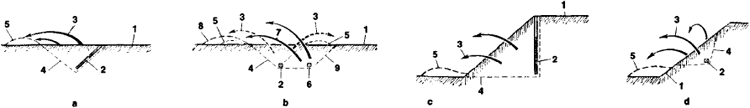# Direction Cosines

Also found in: Dictionary.
The following article is from The Great Soviet Encyclopedia (1979). It might be outdated or ideologically biased.

## Direction Cosines

The direction cosines of a line / are the cosines of the direction angles, where the direction angles α, β, and γ are the angles between a vector along the line / and the positive directions of, respectively, the Ox-axis, Oy-axis, and Oz-axis of a rectangular coordinate system. The direction cosines are related by the equation

cos2α + cos2β + cos2 γ = 1Figure 1. Directional blasting: (a) ejection blasting using blasthole charge, (b) ejection blasting using two chamber charges, (c) collapse blasting using blasthole charge, and (d) collapse blasting using chamber charge; (1) free surface of mass, (2) explosive charge, (3) trajectory of rock fragments, (4) contour of blasting excavation, (5) heap of rock after blasting, (6) explosive charge detonated in second phase, (7) trajectory of fragments from second explosion, (8) heap of rock after second explosion, and (9) contour of excavation after second explosion

The Great Soviet Encyclopedia, 3rd Edition (1970-1979). © 2010 The Gale Group, Inc. All rights reserved.
References in periodicals archive ?
where [u.sub.1], [u.sub.2], and [u.sub.3] are, respectively, the direction cosines of the attitude angels of the platform, and T(t), L(t), and V(t) are, respectively, the transverse, longitudinal, and vertical components of Earth's total magnetic field measured by the vector magnetometer.
where [c.sub.i], i = 1, ..., 3, denotes the compensation coefficients of the permanent magnetic fields and [A.sub.i], i = 1, ..., 3, denotes the variables constituted by the direction cosines.
Additionally, this method can provide automatically paired 2D direction cosine estimates without matching pair and deal with the correlated signals directly.
The half-symmetric Legendre-Chebyshev quadrature sets ([R.sub.n][T.sub.n]) are offered for users, whose set of direction cosines along [xi] axis is different from the sets along other two axes .
So the direction cosines [[lambda].sub.x'], [[lambda].sub.yx'], and [[lambda].sub.z'] of the local coordinate system established at different Gauss point with respect to the global coordinate system are different with each other.
Direction cosines [a.sub.ij] o[x.sub.o] o[x.sub.b] [a.sub.11] = cos [psi] x cos [theta] o[y.sub.b] [a.sub.21] = sin [theta] o[z.sub.b] [a.sub.31] = -sin [psi] x cos [theta] [a.sub.ij] o[y.sub.o] o[x.sub.b] [a.sub.12] = sin[psi] x sin[psi] x cos[phi] x cos[psi] x sin[phi] x sin[theta] o[y.sub.b] [a.sub.22] = cos[phi] x cos[theta] o[z.sub.b] [a.sub.32] = cos[psi] x sin[phi] x sin[psi] x cos[phi] x sin[theta] [a.sub.ij] o[z.sub.o] o[x.sub.b] [a.sub.13] = sin[psi] x cos[phi] + cos[psi] x sin[psi] x sin[theta] o[y.sub.b] [a.sub.23] = -sin[phi] x cos[theta] o[z.sub.b] [a.sub.33] = cos[psi] x cos[phi] - sin[psi] x sin[phi] x sin[theta]
If the direction cosines are positive, the directional radiance is known on the faces W and S and they are unknown on the faces E and N of the (i, j)-cell and also in the centre P.
The main idea of the method uses MUSIC-based procedure to search the true direction cosine estimate from a set of ambiguous candidate estimates.
However, both objects are having deferent type direction cosines (Dcs) and direction ratios (Drs).
Principal components, standard deviation of major and minor axis, direction cosines, and eccentricity of the pattern were derived for each storm.
In space analytic geometry, another new coordinate system can be generated through the initial coordinate system which is rotated one or more times relative to the third coordinate system, and the relationship between the new and old coordinate system can be described with direction cosine. According to this theory, the triaxial acceleration is regarded as a vector, whose three direction cosines are the cosines of three angles between the vector and the three axes, and then the coordinate transformation from the smartphone's coordinate system to the inertial coordinate system will be realized.
where [[tau].sub.xy], [[tau].sub.yz], and [[tau].sub.zx] are shear stress components under the coordinate system oxyz; [l.sub.1], [l.sub.2], and [l.sub.3], respectively, represent direction cosines between x'-, y'-, and z'-axis and x-axis; [m.sub.1], [m.sub.2], and [m.sub.3], respectively, represent direction cosines between x'-, y'-, and z'-axis and y-axis; and [n.sub.1], [n.sub.2], and [n.sub.3], respectively, represent direction cosines between x'-, y'-, and z'-axis and z-axis.

Site: Follow: Share:
Open / Close